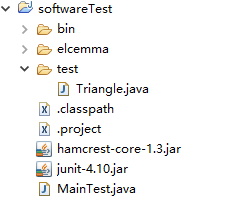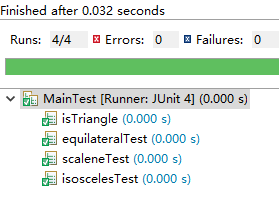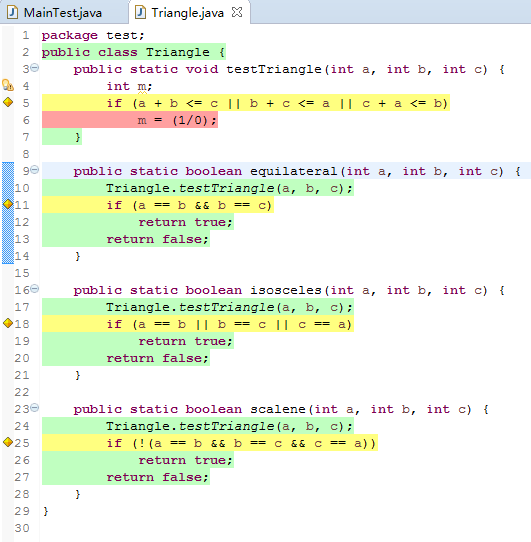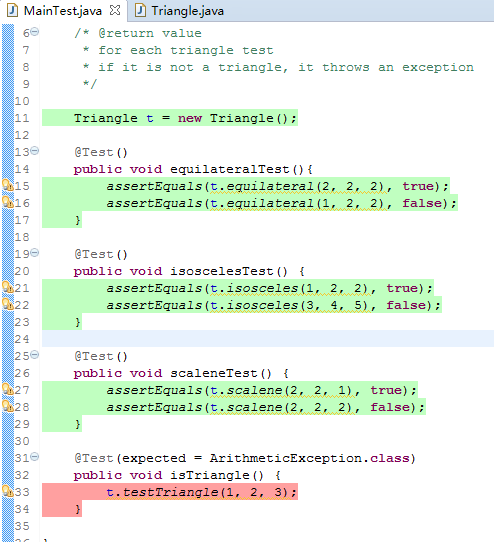• 写一个方法trianglePrint(s){}这个方法输出是afabefabcdef是个等边三角形，要求用递归思想来做，不能有任何for循环谢谢啦publicclassA4Q1umtan42{publicstat...String s = ”abcdef“；写一个方法 trianglePrint...
Strings=”abcdef“；写一个方法trianglePrint(s){}这个方法的输出是afabefabcdef是个等边三角形，要求用递归的思想来做，不能有任何for循环谢谢啦publicclassA4Q1umtan42{publicstat...String s = ”abcdef“；写一个方法 trianglePrint(s){ }这个方法的输出是afabefabcdef是个等边三角形，要求用 递归的思想来做，不能有任何 for 循环 谢谢啦public class A4Q1umtan42{public static void main(String agrs[]){String s1 = "a";String s2 = "abc";String s3 = "abcd";String s4 = "disestablishmentarianism";String s5 = "+-! *# =~ ~= #* !-+";trianglePrint(s1);trianglePrint(s2);trianglePrint(s3);trianglePrint(s4);trianglePrint(s5);}public static void trianglePrint(String s){trianglePrintRecursive(s, 0);}public static void trianglePrintRecursive(String s , int i){//if the length of string is evenif(s.length() % 2 == 0){if(i < s.length()/2){spacesPrint(s , i);System.out.println(s.substring(0, i+1) + s.substring(s.length()-(i+1) , s.length()));trianglePrintRecursive(s , i + 1);}}//if the length is oddelse{String temp = s.concat(" ");trianglePrint(temp);}}public static void spacesPrint(String s , int i){if(i+1 < s.length()/2  ){System.out.print(" ");spacesPrint(s , i + 1);}}}展开
展开全文• 哦，这个简单 import java.util.Scanner; public class Circumference { public static void main(String[] args){ System.out.println("三角形周长计算"); Scanner in = new Scanner(System.in); ...
哦，这个简单  import java.util.Scanner;  public class Circumference {      public static void main(String[] args){          System.out.println("三角形周长计算");          Scanner in = new Scanner(System.in);          System.out.print("请输入三角形第一条边长：");          double a = in.nextDouble();          System.out.print("请输入三角形第二条边长：");          double b = in.nextDouble();          System.out.print("请输入三角形第三条边长：");          double c = in.nextDouble();          if(judge(a,b,c)){              System.out.println("三角形周长为："+calculate(a,b,c));          }          else              System.out.println("你输入的三边不能构成三角形！");      }      private static boolean judge(double a,double b,double c){          if(a+b>c&&a+c>b&&b+c>a)              return true;          else              return false;      }      private static double calculate(double a,double b,double c){          return a+b+c;      }  }
展开全文• 一、简单描述下安装 junit, hamcrest and eclemma过程①当然，有了eclipse软件，...以上可以得到相应版本jar文件，我使用是hamcrest-core-1.3.jar和junit-4.10.jar再在eclipse软件中创建一个项目名叫softw...
一、简单描述下安装 junit, hamcrest and eclemma的过程①当然，有了eclipse软件，安装的过程会显得比较轻松对于安装junit和hamcrest来说需要在官网(或者其它渠道)上面下载标准的jar包即可。以上可以得到相应版本的jar文件，我使用的是hamcrest-core-1.3.jar和junit-4.10.jar再在eclipse软件中创建一个新的项目名叫softwareTest，右键项目的属性，在java build path中将这两个文件引入检验方法可以在运行的run as菜单下看到有Junit Test操作即可。②安装eclemma可以在eclipse软件的帮助菜单下找到eclipse marketplace输入eclemma点击安装即可，检验的方法可以右键这个项目，看到有Coverage As的操作eclemma主要是对测试覆盖进行系统的检测，用明显的颜色来区分代码是否运行到每一行。二、对三角形的问题进行单元测试的编写和coverage覆盖截图检测问题描述：对于设定的整型变量a，b，c，写一些测试，判断它们以这三个构成的三角形(也有可能不能构成三角形)是不是等边、等腰或者斜角三角形ok，老师对于我们的第一次作业要求并不是很高，只是让我们了解了java单元测试相关套件的使用，创建两个文件，一个用于编写三角形文件，一个用于编写测试用例：Triangle.javapackagetest;public classTriangle {public static void testTriangle(int a, int b, intc) {intm;if (a + b <= c || b + c <= a || c + a <=b)m= (1/0);}public static boolean equilateral(int a, int b, intc) {Triangle.testTriangle(a, b, c);if (a == b && b ==c)return true;return false;}public static boolean isosceles(int a, int b, intc) {Triangle.testTriangle(a, b, c);if (a == b || b == c || c ==a)return true;return false;}public static boolean scalene(int a, int b, intc) {Triangle.testTriangle(a, b, c);if (!(a == b && b == c && c ==a))return true;return false;}}MainTest.javaimport staticorg.junit.Assert.assertEquals;importorg.junit.Test;importtest.Triangle;public classMainTest {/*@return value* for each triangle test* if it is not a triangle, it throws an exception*/Triangle t= newTriangle();@Test()public voidequilateralTest(){assertEquals(t.equilateral(2, 2, 2), true);assertEquals(t.equilateral(1, 2, 2), false);}@Test()public voidisoscelesTest() {assertEquals(t.isosceles(1, 2, 2), true);assertEquals(t.isosceles(3, 4, 5), false);}@Test()public voidscaleneTest() {assertEquals(t.scalene(2, 2, 1), true);assertEquals(t.scalene(2, 2, 2), false);}@Test(expected= ArithmeticException.class)public voidisTriangle() {t.testTriangle(1, 2, 3);}}这里我使用了四个测试用例，分别测试是否为等边、等腰、斜角以及是否为三角形(不是三角形抛出异常)，还是比较好理解的，最终测试用例全部通过：测试覆盖也非常简单，点一下，玩一年。。。整个项目代码我已经上传到我的github上面去了
展开全文• 简单工具类写作初衷:由于日常开发经常需要用到很多工具类,经常根据需求自己也比较麻烦 网上好了一些工具类例如commom.lang3或者hutool或者Jodd这样开源工具,但是 发现他们之中虽然设计不错,但是如果我想要使用,...
简单工具类写作初衷:由于日常开发经常需要用到很多工具类,经常根据需求自己写也比较麻烦 网上好了一些工具类例如commom.lang3或者hutool或者Jodd这样的开源工具,但是 发现他们之中虽然设计不错,但是如果我想要使用,就必须要引入依赖并且去维护依赖,有些 甚至会有存在版本编译不通过问题,故此想要写作一个每个类都可以作为独立工具类使用 每个使用者只需要复制该类,到任何项目当中都可以使用,所以需要尊从以下两个原则才能 做到.在此诚邀各位大佬参与.可以把各自用过的工具,整合成只依赖JDK,每个类都能够单独 使用的工具.每个人当遇到业务需求需要使用的时候,只需要到这里单独拷贝一个即可使用. 抛弃传统的需要引入依赖的烦恼.让大家一起来解决你所面临的业务问题吧!介绍遵从两大原则1.绝不依赖JDK以外的源码2.牺牲代码复用性,每个类都必须是单独的组件,绝不互相引用,做到完全解耦package *;import java.text.DecimalFormat;/*** @program: simple_tools* @description: 三角形工具类* @author: ChenWenLong* @create: 2019-06-03 10:29**/public class TriangleUtils {//格式化四舍五入public static final DecimalFormat ROUNDING_OFF = new DecimalFormat("#.00");/*** 功能描述:* 〈获取四舍五入的结果值,并且取得绝对值,解决负数情况〉** @params : [value]* @return : double* @author : cwl* @date : 2019/6/3 15:50*/public static double roundValue(double value){return Math.abs(Double.valueOf(ROUNDING_OFF.format(value)));}/*** 功能描述:* 〈判断是否为三角形〉** @params : [x, y, z]* @return : boolean* @author : cwl* @date : 2019/6/3 11:55*/public static boolean isTriangle(double x,double y,double z){if(x == 0 || y == 0 || z == 0){throw new IllegalArgumentException("This isn't Triangle");}//判断只需要任意两边之和大于第三边就是三角形if(x < y + z || y < x + z || z > x + z){return true;}return false;}/*** 功能描述:* 〈是否是直角三角形〉** @params : [x, y, z]* @return : boolean* @author : cwl* @date : 2019/6/3 11:58*/public static boolean isRightAngleTriangle(double x,double y,double z){//判断是三角形if(isTriangle(x,y,z)){double zEqual = Math.sqrt(Math.pow(x,2)+Math.pow(y,2));double yEqual = Math.sqrt(Math.pow(x,2)+Math.pow(z,2));double xEqual = Math.sqrt(Math.pow(z,2)+Math.pow(y,2));//存在勾股定理成立就是直角三角形if(x == xEqual || y == yEqual || z == zEqual){return true;}}return false;}/*** 功能描述:* 〈是否是等腰直角三角形〉** @params : [x, y, z]* @return : boolean* @author : cwl* @date : 2019/6/3 14:08*/public static boolean isIsoscelesRightTriangle(double x,double y,double z){if(isTriangle(x,y,z)){//是直角三角形,并且存在任意两边相等,即是等腰直角三角形if(isRightAngleTriangle(x,y,z) && (x == y || y == z || x == z)){return true;}}return false;}/*** 功能描述:* 〈是否是30度 60度角的直角三角形〉** @params : [x, y, z]* @return : boolean* @author : cwl* @date : 2019/6/3 14:12*/public static boolean is3060RightTriangle(double x,double y,double z){//是三角形并且是直角三角形if(isTriangle(x,y,z) && isRightAngleTriangle(x,y,z)){//之前的版本存在两个直角边存在两倍关系的情况,所以条件不成立,所以这里修复了限定第三边的情况下去判定斜边是任意直角边的两倍,// 则结果就是30度与60度角的直角三角形boolean xIsHypotenuse = x > y && x > z;boolean yIsHypotenuse = y > x && y > z;boolean zIsHypotenuse = z > y && z > x;if(xIsHypotenuse && (x/2 == y || x/2 == z)){return true;}if(yIsHypotenuse && (y/2 == x || y/2 == z)){return true;}if(zIsHypotenuse && (z/2 == x || z/2 == y) ){return true;}}return false;}/*** 功能描述:* 〈获得三角形的面积〉** @params : [baseLine, highth]* @return : boolean* @author : cwl* @date : 2019/6/3 14:42*/public static double getArea(double baseLine,double highth){if(baseLine == 0 || highth == 0){throw new IllegalArgumentException("This isn't Triangle");}return roundValue((baseLine * highth) / 2);}/*** 功能描述:* 〈获得三角形的周长〉** @params : [x, y, z]* @return : double* @author : cwl* @date : 2019/6/3 14:48*/public static double getPerimeter(double x,double y,double z){if (isTriangle(x,y,z)){return roundValue(x+y+z);}return 0;}/*** 功能描述:* 〈获得直角三角形的周长〉** @params : [x, y]* @return : double* @author : cwl* @date : 2019/6/3 14:49*/public static double getPerimeter(double x,double y){if(x == 0 || y == 0){throw new IllegalArgumentException("This isn't Triangle");}double z = Math.sqrt(Math.pow(x,2)+Math.pow(y,2));return roundValue(x+y+z);}/*** 功能描述:* 〈获得三角函数的sin值,传入读书值〉** @params : []* @return : double* @author : cwl* @date : 2019/6/3 15:31*/public static double getSin(double degree){if(degree >= 179){throw new IllegalArgumentException("Argument isn't more than 180");}if(degree == 0){return 0;}//获得度数占比double proportion = Double.valueOf(ROUNDING_OFF.format(degree / 180));//Math.PI即是πreturn roundValue(Math.PI * proportion);}//=================三角函数 -未完待续========================}
展开全文• 在前面话：昨天给自己做了mbti职业人格测试，得到了自己想要答案，不过果然和计算机一点儿也不搭呢~尽管如此，我认为我还是应该在大学期间认真学好自己专业，因为当初也是自己选嘛，因为不能认输。...
• 编写一个Java应用程序，该程序...此外，Triangle类还有一个boolean型属性，该属性用来判断三个数能够构成一个三角形。完成上述操作后，给Triangle再增加3个方法，分别用来返回3个边sideA, sideB, sideC值。 2.Land.
• 练习1写一个方法void triangle(int a,int b,int c)，判断三个参数是否能构成一个三角形。如果不能则抛出异常IllegalArgumentException，显示异常信息：a,b,c “不能构成三角形”；如果可以构成则显示三角形三个边长...
• 1.写一个方法，用来计算三角形的面积（参数为三个边长，整型，由键盘输入，面积计算用海伦公式： ，p为三角形的半周长 ，使用方法Math.sqrt(),java.lang.Math类里面定义方法），在main方法里面调用该方法，输出面....
• 简单工具类 写作初衷:由于日常开发经常需要用到很多工具类,经常根据需求...甚至会有存在版本编译不通过问题,故此想要写作一个每个类都可以作为独立工具类使用 每个使用者只需要复制该类,到任何项目当中都可以使用...
• 练习1写一个方法void triangle(int a,int b,int c)，判断三个参数是否能构成一个三角形。如果不能则抛出异常IllegalArgumentException，显示异常信息：a,b,c “不能构成三角形”；如果可以构成则显示三角形三个边长...
• java实现简单工厂模式 --------记录人生第次博客 ...实验要求：1、有如下场景：现有绘图工具，能够支持圆形、三角形、正方形等绘图，每图形都有绘图和擦除两方法，请结合简单工厂模式实现该工具...设计模式
• 一个做界面的我们大概都知道了，这一次我们没有复选框文本框，就是一个简单的画面，选择他的框的长宽，然后，流式布局。界面的代码，参照上个博客就好。 所以本次我们的任务分为几个步骤完成： 1.创建窗体（咱们...画布
• 练习1写一个方法void triangle(int a,int b,int c)，判断三个参数是否能构成一个三角形。如果不能则抛出异常IllegalArgumentException，显示异常信息：a,b,c “不能构成三角形”；如果可以构成则显示三角形三个边长...
• OpenGL ES 3.0 上的一个三角形例子，网上可以下载到android skd 版（java）和 android ndk (c&c++版） 为了了解一下JNI，于是了如下小程序。 这个例子是使用jni, java中调用c中代码完成三角形渲染, 其中...
• 打开jmeter，在测试计划中右键，添加一个线程组。...到此，我们可以开始写一个小小用例了。 第一种写法： 点击上面的三角形开始运行。 点击查看结果树，查看响应结果： 第二种写法： 查看结果树，响应结果： 在...
• 打开jmeter，在测试计划中右键，添加一个线程组。...到此，我们可以开始写一个小小用例了。 第一种写法： 点击上面的三角形开始运行。 点击查看结果树，查看响应结果： 第二种写法： 查看结果树，响应结果： 在...
• HasStatic.java 一个简单的拥有静态成员的类 hideMember_1.java 成员隐藏示例1 hideMember_2.java 成员隐藏示例2 hideMember_3.java 成员隐藏示例3 hideMember_4.java 成员隐藏示例4 hideMember_5.java 成员...
• 一、KNN手写识别原理 在下图中，要判断绿色圆归属为哪个类（红三角形还是蓝...KNN使用是欧氏距离原理：（可以把图形当作一个点）计算两个点间距离 这个是二维，如果是三维或者多维欧氏距离： 两个n维...手写体识别
• 今天群里有个人问，怎样用增则表达式匹配三角形的三边，其实只是要匹配三个数字而已，如 301 402 503 开始认为很简单，我就一个 "(([1-9]\\d?)\\s){2}$2" 结果他说错了，我感觉很奇怪，于是自己打开电脑试了试，...正则表达式 • 绘制一个三角形 正如我们学习Java、C++等编程语言时...接下来我们就按照上述所说渲染过程，讲解一下如何通过OpenGL ESAPI在Android手机上显示出一个三角形。 在Demo中我们创建一个 TriangleActivity&...三维图形绘制 • 今天群里有个人问，怎样用增则表达式匹配三角形的三边，其实只是要匹配三个数字而已，如 301 402 503 开始认为很简单，我就一个 "(([1-9]\\d?)\\s){2}$2" 结果他说错了，我感觉很奇怪，于是自己打开电脑试了试，...
• 4. 高中几何课曾介绍过勾股定理(即直角三角形三条边长度之间关系)：也可以成以下形式这表达式中大部分运算符在第三章中已经介绍过，唯一没有出现是开平方操作，而这操作可以通过调用标准函数Math.sqrt...class import integer
• 最近在上算法课，上课老师讲到了维诺图，自己觉得很有意思就研究了一下，并用java写一个简单的se程序用来显示维诺图和Delaunay三角形还有凸包。 首先介绍一下凸包，凸包在数学里是很常见的，给定一些点，然后找出...
• Delaunay三角网，了用半天，调试BUG用了2天……醉了。基本思路比较简单，但效率并不是很快。1. 先生成一个凸包；...每插入一个点 ptA，都要判断被插入点存在于 Triangles 集合哪个三角形(trianA...
• 1-5 在Eclipse开发第一个Java程式 23 1-6 在Eclipse开发第一个Java视窗程式-显示影像 26 1-7 在Eclipse开发视窗程式-slider控制元件 34 1-8 在Eclipse开发视窗程式-按钮控制元件 39 1-9 好用Eclipse热键 41 第2章 ...
• 学习java程序，与学习python有大同小异之处，都是编程...我曾想写个代码多么容易，但它是必须进行一步，让你了解程序是怎样运行。 三，敲打简单代码 比如:直角三角形，爱心，个人信息，史努比(偷偷告诉你们，当...
• 一个大神的图形识别，能识别矩形三角形和圆形，虽然识别率不高但是简单的识别应该满足了opencv# java简单的写一个三角形java 订阅